Try NerdPal! Our new app on iOS and Android

# Find the derivative $\frac{d}{dx}\left(x^2\right)$ using the power rule

## Related Videos

Go!
Go!
1
2
3
4
5
6
7
8
9
0
a
b
c
d
f
g
m
n
u
v
w
x
y
z
.
(◻)
+
-
×
◻/◻
/
÷
2

e
π
ln
log
log
lim
d/dx
Dx
|◻|
θ
=
>
<
>=
<=
sin
cos
tan
cot
sec
csc

asin
acos
atan
acot
asec
acsc

sinh
cosh
tanh
coth
sech
csch

asinh
acosh
atanh
acoth
asech
acsch

### Videos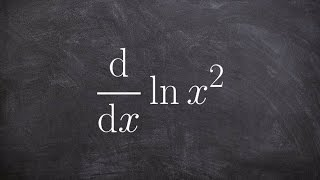### Calculus - Using the power rule of logarithms to take the derivative of a natural log, d(ln(x^2))/dx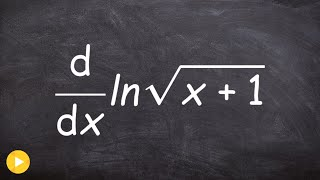### Calculus - Using power rule with square root to take derivative on a logarithm, d(ln(sqrt(x+1)))/dx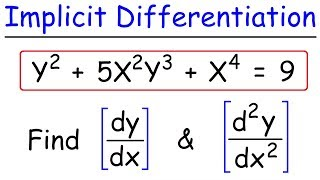### Implicit Differentiation - Find The First &amp; Second Derivatives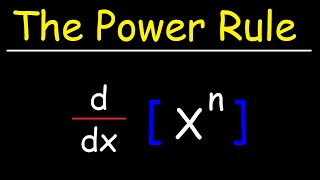### The Power Rule For Derivatives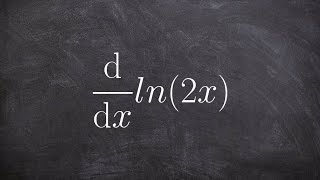### Calculus - Find the derivative of natural logarithm using product property, d(ln(2x))/dx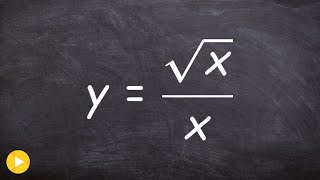SnapXam A2

### beta Got another answer? Verify it!

Go!
1
2
3
4
5
6
7
8
9
0
a
b
c
d
f
g
m
n
u
v
w
x
y
z
.
(◻)
+
-
×
◻/◻
/
÷
2

e
π
ln
log
log
lim
d/dx
Dx
|◻|
θ
=
>
<
>=
<=
sin
cos
tan
cot
sec
csc

asin
acos
atan
acot
asec
acsc

sinh
cosh
tanh
coth
sech
csch

asinh
acosh
atanh
acoth
asech
acsch

$\frac{d}{dx}\left(x^2\right)$

### Main topic:

Power Rule for Derivatives

~ 0.03 s